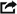If you really want to do the job right, you should convert the co-ordinates to galactic co-ords. This is where the X-Y plane is the plane of the Galaxy, which you might agree is a tad less parochial than the earth centered ones. The positive X-axis points at the galactic center.

Some astronomers in galactic structure use +Y as the coreward axis, while +X points anti-spinward. See details here.

## Coordinate TransformWhat you have to do is perform a coordinate transform to convert from equatorial to galactic co-ords. This means you'll take the equatorial x, y, and z coordinates and feed them into three equations. Out will pop the galactic x,y,z.

### Epoch 1950.0

If your star data is in epoch 1950.0 (like the Gliese data), use the equations below from Bruce Webster's New Perspectives on Nearby Stars, published in July 1985 issue of BYTE Magazine.

Xg = -(0.0672 * X) - (0.8727 * Y) - (0.4835 * Z)

Yg = (0.4927 * X) - (0.4504 * Y) + (0.7445 * Z)

Zg = -(0.8676 * X) - (0.1884 * Y) + (0.4602 * Z)

They seem to work, I fed in the co-ordinates of the galactic center and got a point about 10 kiloparsecs on the positive X axis. (RA 17h42m4s, DEC -28°55', DIST 27,000 light years, in case you were wondering.)

Late breaking news, the latest (2019) measured distance to the center of the galaxy is 26,660 light-years. Specifically 8178 parsecs ± 13stat. ± 22sys.

### Epoch 2000.0

If your star data is in epoch 2000.0, use the following equations:

Xg = -(0.0550*X) -(0.8732*Y) - (0.4839*Z)

Yg = (0.4940*X) - (0.4449*Y) + (0.7470*Z)

Zg = -(0.8677*X) - (0.1979*Y) + (0.4560*Z)

These equations are based on the following co-ordinates:

Galactic center: RA = 17h45.6m; Dec =-28°56.3'

Galactic north pole: RA = 12h51.4m; Dec = +27°07.7'

These equations are curtesy of William Sandberg. For those who are interested in the mathematical derivation of those equations, go here. The derivation was also independently worked out by Andrew Goddard, who has also written a nice set of astronomical online calcuators. (I would have posted Andrew's derivation, except I lost both it and his email address the last time my hard drive crashed.)

## Heliocentric Co-ordinates

And if you really want to be anal-retentive, Sol is currently about 50 light years above the plane of the galaxy. So add 50 light years (or 15.3 parsecs) to the galactic Z co-ord. (a 1995 survey by Humphreys, Larsen, and Cohen gave values of 8, 15.5 and 14 parsecs depending on the wavelength used for the survey. Take your pick.)

Not so fast, says Chris Lawson:

Anyway, for true non-helocentric co-ords, first you have to choose what defines the X-axis. For purposes of illustration, let's say that the negative X-axis points at the projection of Andromeda Galaxy onto the galactic plane.

If you start with equitorial co-ords, and use the equations at the top of this page, you will have heliocentric galactic co-ords. (Sun at origin, x-y plane is galactic plane, positive X-axis points at galactic center.)

Subtract 8,300 parsecs (27,000 light years) from each X co-ord and add 15 parsecs (50 light years) to each Z co-ord to get Sun indexed galactic co-ords. (Galactic center at origin, x-y plane is galactic plane, negative X-axis points at the Sun.)

Xs = Xg - 8,300

Ys = Yg

Zs = Zg + 15

Say the Andromeda Galaxy is at Xa, Ya, Za. The projection of the Andromeda Galaxy on the x-y plane is at Xa, Ya, 0.

To transform all the co-ordinates so that the negative X-axis points at the Andromeda Galaxy's projection, do the following:

R = sqrt( Xa2 + Ya2)

X' = (-Xs * (Ya/R)) + (Ys * (Xa/R))

Y' = (-Xs * (Xa/R)) + (Ys * (Ya/R))

Z' = Zs

(Thanks to Erich Schneider for the above equations.)

If you want to go into more mathematical detail, be sure to check out James Shuster's incredible SGC page. Very well done, and much more mathematically rigorous (translation: most of it is over my head...) He also has some notes on interstellar navigation.

## Atomic Rockets notices

Welcome to the improved 3-D Starmaps!